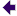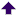### Growth table

عربي Arabic - 汉语 Chinese - Deutsch - English - español - français - Italiano - 日本人 Japanese - Nederlands - Português - Русский Russian
Some pages must still be translated - who will help?
The table shows the total increase after a given number of years at various constant growth rates.
Cumulative growth [growth factor] = total increase in quantities after a given number of years at a certain annual growth rate:
• Cumulative growth = total growth in percent = the percentage by which the quantity will have increased
• [Growth factor] = by how many times the quantity will have increased
Example: With an initial level of 300 and an increase of 50 per cent, the final level will be 450. The growth factor is then 1.5 times. Further examples: see at the bottom of the table.
•5 years 10 years 15 years 20 years 25 years 50 years 75 years 100 years
0,5 %     + 2,53 % + 5,11 % + 7,77 % + 10,5 % + 13,3 % + 28,3 % + 45,4 % + 64,7 %
1 %     + 5,1 % + 10,5 % + 16,1 % + 22,0 % + 28,2 % + 64,5 % + 111 %
[ 2,1 x ]
+ 170 %
[ 2,7 x ]
1,5 %     + 7,73 % + 16,1 % + 25,0 % + 34,9 % + 45,1 % + 111 %
[ 2,1 x ]
+ 205 %
[ 3 x ]
+ 343 %
[ 3,4 x ]
2 %     + 10,4 % + 21,9 % + 34,6 % + 48,6 % + 64,1 % + 169 %
[ 2,7 x ]
+ 342 %
[ 4,4 x ]
+ 625 %
[ 7,2 x ]
2,5 %     + 13,1 % + 28,0 % + 44,8 % + 63,9 % + 85,4 % + 244 %
[ 3,4 x ]
+ 573 %
[ 6,4 x ]
+ 1081 %
[ 11,8 x ]
3 %     + 15,9 % + 34,4 % + 55,8 % + 80,6 % + 109 %
[ 2,1 x ]
+ 338 %
[ 4,4 x ]
+ 818 %
[ 9,2 x ]
+ 1822 %
[ 19,2 x ]
4 %     + 22 % + 48 % + 80 % + 119 %
[ 2.2 x ]
+ 167 %
[ 2.7 x ]
+ 611 %
[ 7.1 x ]
+ 1795 %
[ 18.9 x ]
+ 4905 %
[ 50.5 x ]
5 %     + 28 % + 63 % + 108 %
[ 2,1 x ]
+ 165 %
[ 2,6 x ]
+ 239 %
[ 3,4 x ]
+ 1047 %
[ 11 x ]
+ 3783 %
[ 39 x ]
+ 13050 %
[ 131 x ]
6 %     + 34 % + 79 % + 140 %
[ 2,4 x ]
+ 221 %
[ 3,2 x ]
+ 329 %
[ 4,3 x ]
+ 1742 %
[ 18 x ]
+ 7806 %
[ 79 x ]
+ 32830 %
[ 329 x ]
7 %     + 40 % + 97 % + 176 %
[ 2,8 x ]
+ 287 %
[ 3,9 x ]
+ 443 %
[ 5,4 x ]
+ 2846 %
[ 29 x ]
+ 15888 %
[ 160 x ]
+ 86672 %
[ 868 x ]
8 %     + 47 % + 116 %
[ 2,2 x ]
+ 217 %
[ 3,2 x ]
+ 366 %
[ 4,7 x ]
+ 585 %
[ 6,8 x ]
+ 4590 %
[ 47 x ]
+ 32020 %
[ 321 x ]
+ 219900 %
[ 2200 x ]
9 %     + 54 % + 137 %
[ 2,4 x ]
+ 264 %
[ 3,6 x ]
+ 460 %
[ 5,6 x ]
+ 762 %
[ 8,6 x ]
+ 7336 %
[ 74 x ]
+ 64019 %
[ 641 x ]
+ 552804 %
[ 5529 x ]
10 %     + 61 % + 159 %
[ 2,6 x ]
+ 318 %
[ 4,2 x ]
+ 573 %
[ 6,7 x ]
+ 983 %
[ 10,8 x ]
+ 11839 %
[ 118 x ]
+ 127090 %
[ 1272 x ]
+ 1379961 %
[ 13801 x ]
Examples:

• If the economy would have an annual economic growth of 3 %, as desired by economists, then production and consumption would in 25 years (one generation) have more than doubled (1,0325 = 2,0938 = 2,1×). We would have the double amount of cheese, cars, factories, hospitals, etc., provided the production and consumption pattern remains the same. But even if the pattern would change, the total Gross Domestic Product would still be more than double. The GDP is the money value of all that is produced and represents all goods and services. One should also know that so-called "services" are mostly very material and resource consuming, such as transportation, etc., and even banks
• At a growth rate of 3 %, a country will in four generations have 19 times more of everything.
At the end of this century China would have 2200 times more, at their 2005 growth rate of 8 %.

How can we continue to increase production and consumption, if a country, in fact the planet, has a fixed size and the amount of resources decreases?
So-called "qualitative growth" is no answer because all growth is expressed in money and represents resources. More quality requires more money and resources.

• عربي Arabic - 汉语 Chinese - Deutsch - English - español - français - Italiano - 日本人 Japanese - Nederlands - Português - Русский Russian
Some pages must still translated - who will help?:home | index |growth | forum | feedback |ecoglobe - since 1997
5227-6916org